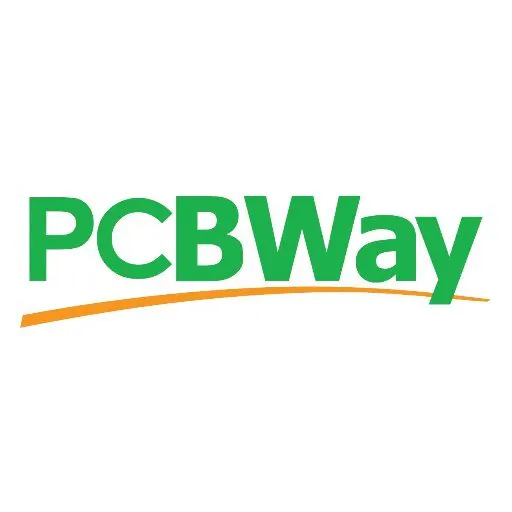Programmable Timer for Activation of Devices - Part II

In this the second part you'll learn how to implement the timer and function to program the hour to activate the device.

IntermediateFull instructions provided2 hours707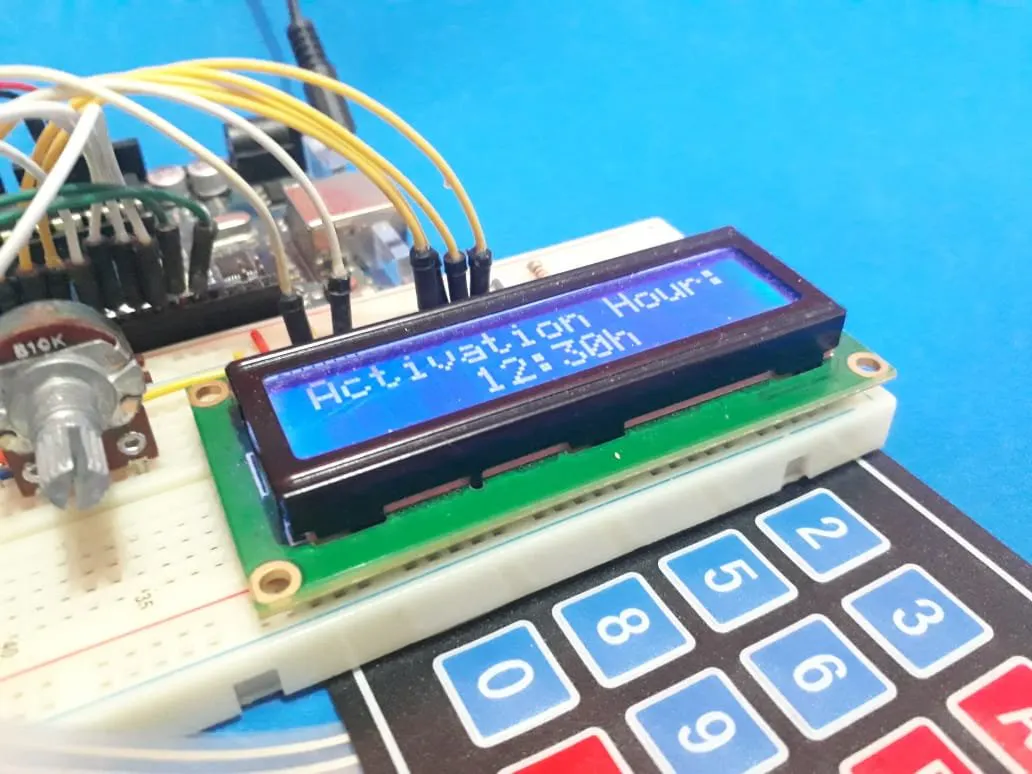Things used in this project

Hardware components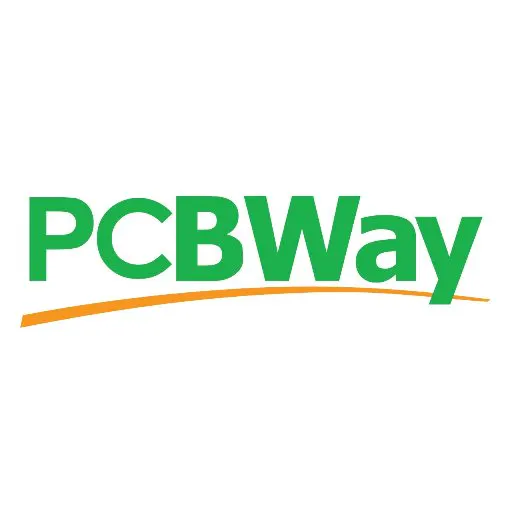PCBWay Custom PCB
×1
×1
 Real Time Clock - DS1307
×1
 Potentiometer 10kR - UsinaInfo Store Brazil
×1Arduino UNO & Genuino UNO
×1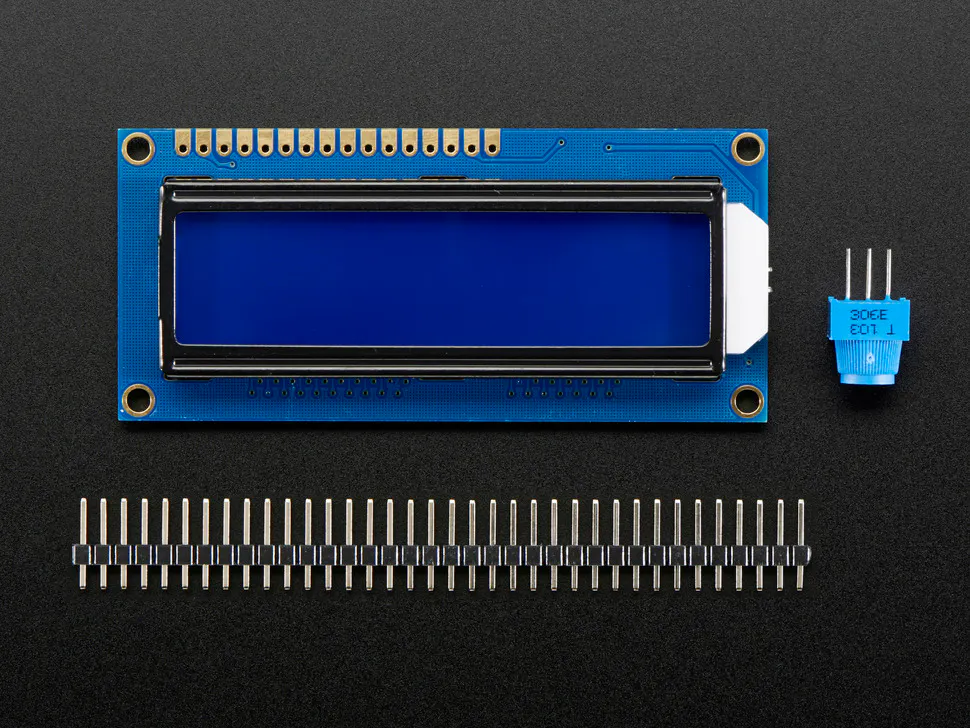Adafruit Standard LCD - 16x2 White on Blue
×1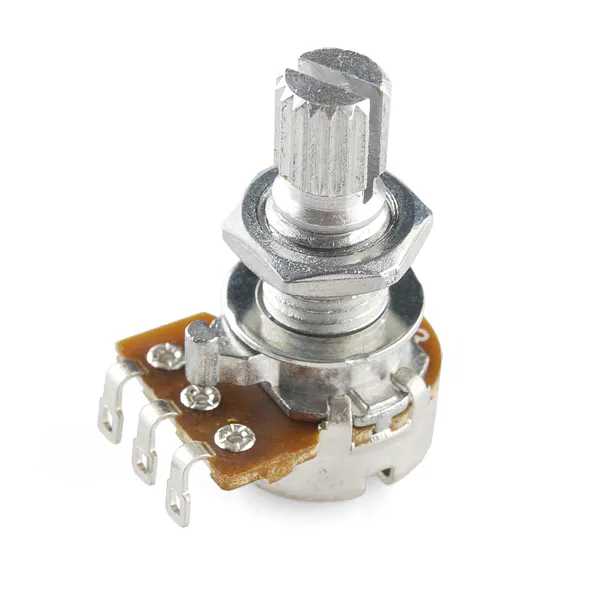Rotary potentiometer (generic)
×1Jumper wires (generic)
×1

Software apps and online servicesArduino IDE

Schematics

Schematic Circuit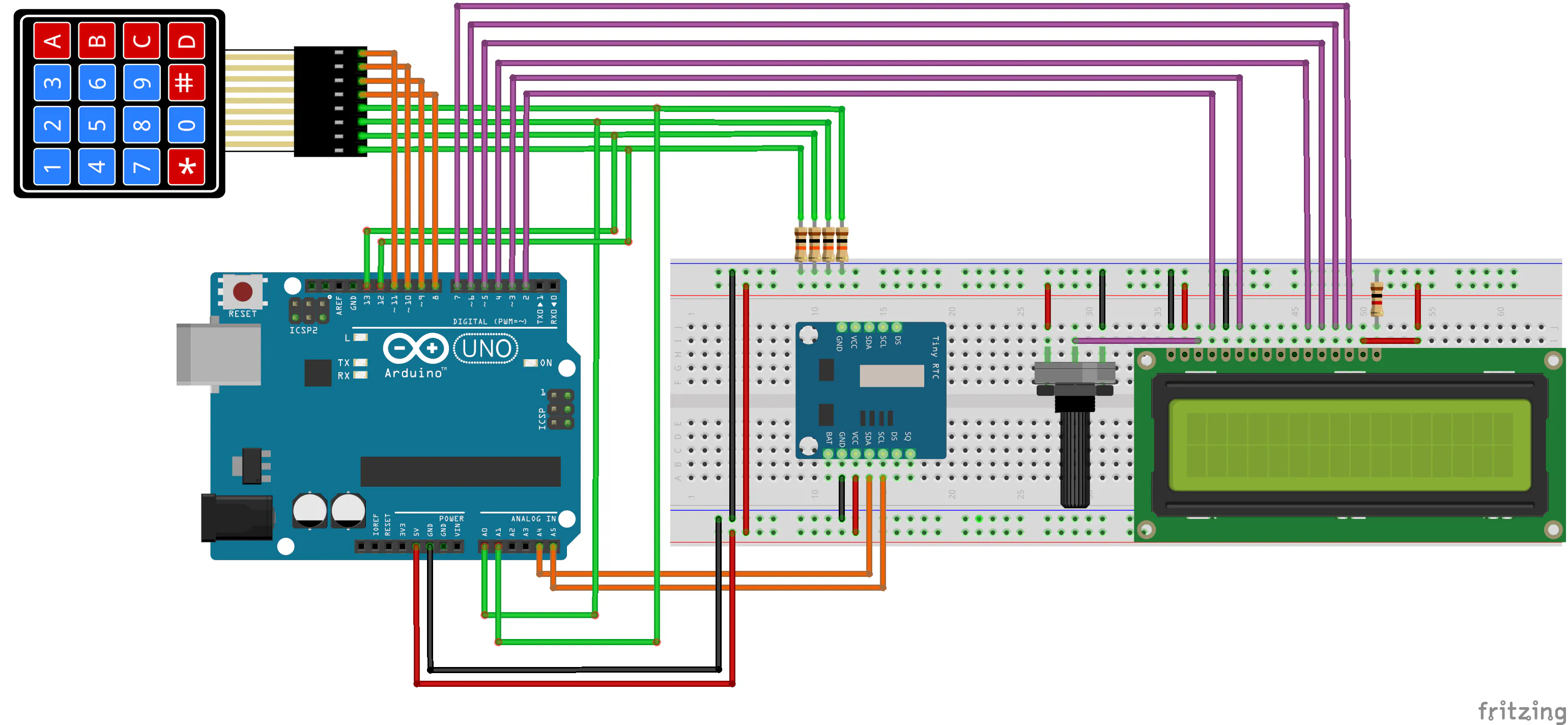Code

Code for Second Part of the System

Arduino
#include <DS1307.h>
#include <Wire.h>
#include <EEPROM.h>
#include <LiquidCrystal.h>

#define MEMORY 100

const int rs = 2, en = 3, d4 = 4, d5 = 5, d6 = 6, d7 = 7;
LiquidCrystal lcd(rs, en, d4, d5, d6, d7);

char tempo = "";
char data = "";

int DataTime;

byte SegAnt = 0, SegAtual = 0;

void ActivationHour(void);

void setup()
{
Serial.begin(9600);
DS1307.begin();
//Config pins to control columns of the matrix keyboard
for(int key = 8; key < 12; key++)
{
pinMode(key, OUTPUT);
}

//Config pins to read lines of the matrix keyboard
for(int key = 12; key < 16; key++)
{
pinMode(key, INPUT);
}

for(int key = 8; key < 12; key++)
{
digitalWrite(key, LOW);
}

lcd.begin(16,2);

lcd.clear();
lcd.setCursor(4,0);
lcd.print("Invalid");
lcd.setCursor(6,1);
lcd.print("Hour");
delay(30000);
}

void loop()
{
{
EEPROM.write(MEMORY, 73);
lcd.clear();
ActivationHour();
lcd.clear();
}

DS1307.getDate(DataTime);
SegAtual = DataTime;

{
lcd.clear();
ActivationHour();
lcd.clear();
}

for(byte i = 1; i < 5; i++)
{
}

if(abs(SegAtual - SegAnt) >= 1)
{
sprintf(tempo, "%02d:%02d:%02d", DataTime, DataTime, DataTime);
sprintf(data, "%02d/%02d/%02d", DataTime, DataTime, DataTime);

lcd.setCursor(4,1);
lcd.print(tempo);
lcd.setCursor(4,0);
lcd.print(data);
SegAnt = SegAtual;
}
}

{
byte teclado = {
{1,2,3,10},
{4,5,6,11},
{7,8,9,12},
{14,0,15,13}};

byte coluna = {8,9,10,11};
byte linha  = {12,13,14,15};
bool tecla;

byte digito_tecla = 16;

for(byte y = 0; y <= 3; y++)
{
digitalWrite(coluna[y], LOW);
}

for(byte y = 0; y <= 3; y++)
{

digitalWrite(coluna[y], HIGH);

for(byte x = 0; x <= 3; x++)
{

if(tecla == 1)
{
return digito_tecla;
}
}

digitalWrite(coluna[y], LOW);
}

return digito_tecla;
}

{
int times;
bool controle = 0;
bool EstadoSobe = 0, EstadoDesce = 0;
byte cont = 0;
byte number = 0;
int digitos;
byte PosCursor = 0;
byte t = 0;
bool Validate = 0;
char tempo = "";
char data = "";

digitos = times/10; //Armazena a Dezena das Horas
digitos = times%10; //Armazena a Unidade das Horas
digitos = times/10; //Armazena a Dezena dos Minutos
digitos = times%10; //Armazena a Unidade dos Minutos

do
{

lcd.setCursor(0,0);
lcd.print("                ");
lcd.setCursor(2,0);
lcd.setCursor(0,1);
lcd.print("                ");
lcd.setCursor(5,1);
lcd.print(tempo);
PosCursor = 5;

do
{
delay(100);
if( (number >= 0 && number <= 9) && (controle == 0) && (cont < 4) )
{
digitos[cont] = number;
cont++;
controle = 1;
lcd.setCursor(PosCursor,1);
lcd.print(number);
PosCursor++;
if(cont == 2 || cont == 4)
{
PosCursor = PosCursor + 1;
}
}
if(number == 16 && controle == 1)
{
controle = 0;
}
if(number == 12)
{
for(cont = 0; cont < 4; cont++)
{
digitos[cont] = 0;
}
lcd.setCursor(0,1);
lcd.print("                ");
lcd.setCursor(5,1);
lcd.print("00:00h");
PosCursor = 5;
cont = 0;
for(int i = 4; i < 6; i++)
{
times[i] = 0;
}
}

}while(number != 13);

times = (digitos*10) + digitos;
times = (digitos*10) + digitos;

if((times < 0 || times > 23) || (times < 0 || times > 59))
{
lcd.clear();
lcd.setCursor(3,0);
lcd.print("Invalid");
lcd.setCursor(4,1);
lcd.print("Hour");

delay(2000);
Validate = 1;

lcd.clear();
lcd.setCursor(0,0);
lcd.print("                ");
lcd.setCursor(1,0);
lcd.setCursor(0,1);
lcd.print("                ");
lcd.setCursor(5,1);
lcd.print("00:00h");
sprintf(tempo, "%02d:%02dh", 0, 0);
PosCursor = 5;
cont = 0;

for(int i = 0; i < 4; i++)
{
times[i] = 0;
}

}
}while(Validate == 1);

do
{

delay(200);

}while(number != 16);
/*----------------------------------------Date Configuration-----------------------------------------------*/

PosCursor = 4;

do
{
//Transformacao dos digitos da data para dezenas e unidades
digitos = times/10; //Armazena a Dezena da Data
digitos = times%10; //Armazena a Unidade da Data
digitos = times/10; //Armazena a Dezena do Mes
digitos = times%10; //Armazena a Unidade do Mes
digitos = times/10; //Armazena a Dezena do Ano
digitos = times%10; //Armazena a Unidade do Ano
sprintf(data, "%02d/%02d/%02d", times, times, times);

lcd.setCursor(0,0);
lcd.print("                ");
lcd.setCursor(2,0);
lcd.setCursor(0,1);
lcd.print("                ");
lcd.setCursor(4,1);
lcd.print(data);
PosCursor = 4;
cont = 0;

do
{

delay(100);

if( (number >= 0 && number <= 9) && (controle == 0) && (cont < 6) )
{
digitos[cont] = number;
cont++;
controle = 1;
lcd.setCursor(PosCursor,1);
lcd.print(number);
PosCursor++;
if(cont == 2 || cont == 4)
{
PosCursor = PosCursor + 1;
}
}

if(number == 16 && controle == 1)
{
controle = 0;
}

if(number == 12)
{
for(cont = 0; cont < 6; cont++)
{
digitos[cont] = 0;
}
lcd.setCursor(0,1);
lcd.print("                ");
lcd.setCursor(4,1);
lcd.print("00/00/00");
PosCursor = 4;
cont = 0;
}
}while(number != 13);

times = (digitos*10) + digitos; //Transformando os numeros lidos para data do mes em dois dígitos
times = (digitos*10) + digitos; //Transformando os numeros lidos para mes em dois dígitos
times = (digitos*10) + digitos; //Transformando os numeros lidos para ano em dois dígitos

if((times <= 0 || times > 31) || (times <= 0 || times > 12) || times <= 0)
{
lcd.clear();
lcd.setCursor(3,0);
lcd.print("Invalid");
lcd.setCursor(4,1);
lcd.print("Date");
delay(2000);
Validate = 1;
lcd.clear();
lcd.setCursor(0,0);
lcd.print("                ");
lcd.setCursor(2,0);
lcd.setCursor(0,1);
lcd.print("                ");
lcd.setCursor(3,1);
lcd.print("00/00/00");
PosCursor = 5;
cont = 0;

for(byte i = 0; i < 3; i++)
{
times[i] = 0;
}

}
}while(Validate == 1);
do
{
delay(200);
}while(number != 16);

lcd.clear();

DS1307.setDate(times,times,times,times,times,times,00);//year,month,date of month, day of week,hour,minutes,second
}

void ActivationHour(void)
{
int times;
bool controle = 0;
bool EstadoSobe = 0, EstadoDesce = 0;
byte cont = 0;
byte number = 0;
int digitos;
byte PosCursor = 0;
bool Validate = 0;
lcd.clear();

for(byte i = 0; i < 6; i++)
{
digitos[i] = 0;
}
do
{
lcd.setCursor(0,0);
lcd.print("                ");
lcd.setCursor(0,0);
lcd.print("Activation Hour:");
lcd.setCursor(0,1);
lcd.print("                ");
lcd.setCursor(5,1);
lcd.print("00:00h");
PosCursor = 5;
do
{
delay(100);
if( (number >= 0 && number <= 9) && (controle == 0) && (cont < 4) )
{
digitos[cont] = number;
cont++;
controle = 1;
lcd.setCursor(PosCursor,1);
lcd.print(number);
PosCursor++;
if(cont == 2 || cont == 4)
{
PosCursor = PosCursor + 1;
}
}
if(number == 16 && controle == 1)
{
controle = 0;
}
if(number == 12)
{
for(cont = 0; cont < 4; cont++)
{
digitos[cont] = 0;
}

lcd.setCursor(0,1);
lcd.print("                ");
lcd.setCursor(5,1);
lcd.print("00:00h");
PosCursor = 5;
cont = 0;
for(int i = 4; i < 6; i++)
{
times[i] = 0;
}
}
}while(number != 13);
times = (digitos*10) + digitos;
times = (digitos*10) + digitos;

if((times < 0 || times > 23) || (times < 0 || times > 59))
{
lcd.clear();
lcd.setCursor(3,0);
lcd.print("Invalid");
lcd.setCursor(4,1);
lcd.print("Hour");
delay(2000);
Validate = 1;

lcd.clear();
lcd.setCursor(0,0);
lcd.print("                ");
lcd.setCursor(1,0);
lcd.print("Activation Hour:");
lcd.setCursor(0,1);
lcd.print("                ");
lcd.setCursor(5,1);

lcd.print("00:00h");
sprintf(tempo, "%02d:%02dh", 0, 0);

PosCursor = 5;
cont = 0;

for(cont = 0; cont < 4; cont++)
{
digitos[cont] = 0;
}

}
}while(Validate == 1);

EEPROM.write(1, times);
EEPROM.write(2, times);

do
{
delay(200);
}while(number != 16);
}

Credits

Silícios Lab

49 projects • 100 followers
Hello, I'm Diego Moreira, founder of the Silícios LAB and I love program microcontrollers and works with electronic projects.

PCBWay

50 projects • 86 followers
We are a pcb prototype and assembly manufacturer to help creators build projects. As low as \$5/10pcs and 3 days delivery time.